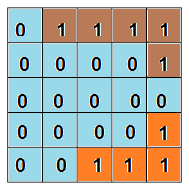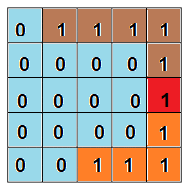New update is available. Click here to update.

# Shortest Bridge

Last Updated: 25 Jan, 2021
Difficulty: Moderate

## PROBLEM STATEMENT

#### For example#### For example#### Tony is busy assembling all the avengers, so he called you to solve this problem.

##### Input Format
``````The first line of input contains an integer 'T' representing the number of test cases. Then the 'T' test cases are as follows.

The first line of each test case contains two single-spaced integers ‘N’ and ‘M’, representing the number of rows and columns of the 2-D array, respectively.

For the next 'N' lines, each line contains 'M' space-separated integers (0 or 1), where 0 denotes the water and 1 denotes the land.
``````
##### Output Format:
``````For each test case, print the length of the shortest bridge which connects the two islands.

The output for each test case is printed in a separate line.
``````
##### Note:
``````You do not need to print anything, it has already been taken care of. Just implement the given function.
``````
##### Constraints:
``````1 <= T <= 5
1 <= N, M <= 100
0 <= ARR[i][j] <= 1

Where ‘ARR[i][j]’ is the value of the elements of the 2-D array.

Time Limit: 1 sec.
``````